# JP2012189752A - Display device - Google Patents

## Info

Publication number
JP2012189752A
JP2012189752A JP2011052568A JP2011052568A JP2012189752A JP 2012189752 A JP2012189752 A JP 2012189752A JP 2011052568 A JP2011052568 A JP 2011052568A JP 2011052568 A JP2011052568 A JP 2011052568A JP 2012189752 A JP2012189752 A JP 2012189752A
Authority
JP
Japan
Prior art keywords
gate
voltage
circuit
signal
circuits
Prior art date
Legal status (The legal status is an assumption and is not a legal conclusion. Google has not performed a legal analysis and makes no representation as to the accuracy of the status listed.)
Withdrawn
Application number
JP2011052568A
Other languages
Japanese (ja)
Inventor
Takahiro Ochiai
Mitsuru Goto

Original Assignee
Japan Display East Co Ltd

Priority date (The priority date is an assumption and is not a legal conclusion. Google has not performed a legal analysis and makes no representation as to the accuracy of the date listed.)
Filing date
Publication date
Application filed by Japan Display East Co Ltd, 株式会社ジャパンディスプレイイースト filed Critical Japan Display East Co Ltd
Priority to JP2011052568A priority Critical patent/JP2012189752A/en
Publication of JP2012189752A publication Critical patent/JP2012189752A/en
Withdrawn legal-status Critical Current

• 230000000875 corresponding Effects 0.000 claims abstract description 40
• 239000011159 matrix materials Substances 0.000 claims abstract description 5
• 239000003990 capacitor Substances 0.000 claims description 10
• 230000001276 controlling effects Effects 0.000 claims description 8
• 230000003071 parasitic Effects 0.000 abstract description 19
• 230000004048 modification Effects 0.000 description 16
• 238000006011 modification reactions Methods 0.000 description 16
• 239000000758 substrates Substances 0.000 description 14
• 238000010586 diagrams Methods 0.000 description 9
• 239000004973 liquid crystal related substances Substances 0.000 description 7
• 241001671220 Stachyuraceae Species 0.000 description 6
• 230000001808 coupling Effects 0.000 description 4
• 238000010168 coupling process Methods 0.000 description 4
• 238000005859 coupling reactions Methods 0.000 description 4
• 230000002457 bidirectional Effects 0.000 description 3
• 230000000694 effects Effects 0.000 description 3
• 239000010410 layers Substances 0.000 description 3
• 239000000463 materials Substances 0.000 description 3
• 239000004065 semiconductors Substances 0.000 description 3
• 230000001629 suppression Effects 0.000 description 2
• 101710047752 ABCB23 Proteins 0.000 description 1
• 101710070464 SOT15 Proteins 0.000 description 1
• 101710020975 hst2 Proteins 0.000 description 1
• 230000001681 protective Effects 0.000 description 1
• 101710054351 sta-2 Proteins 0.000 description 1

## Images

•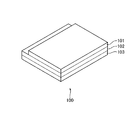•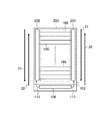••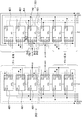•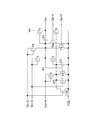•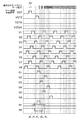•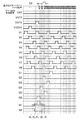•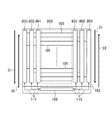•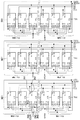•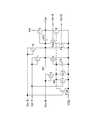•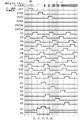•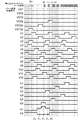•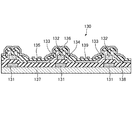•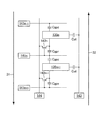•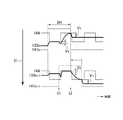•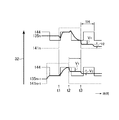## Classifications

• GPHYSICS
• G09EDUCATION; CRYPTOGRAPHY; DISPLAY; ADVERTISING; SEALS
• G09GARRANGEMENTS OR CIRCUITS FOR CONTROL OF INDICATING DEVICES USING STATIC MEANS TO PRESENT VARIABLE INFORMATION
• G09G3/00Control arrangements or circuits, of interest only in connection with visual indicators other than cathode-ray tubes
• G09G3/20Control arrangements or circuits, of interest only in connection with visual indicators other than cathode-ray tubes for presentation of an assembly of a number of characters, e.g. a page, by composing the assembly by combination of individual elements arranged in a matrix no fixed position being assigned to or needed to be assigned to the individual characters or partial characters
• G09G3/34Control arrangements or circuits, of interest only in connection with visual indicators other than cathode-ray tubes for presentation of an assembly of a number of characters, e.g. a page, by composing the assembly by combination of individual elements arranged in a matrix no fixed position being assigned to or needed to be assigned to the individual characters or partial characters by control of light from an independent source
• G09G3/36Control arrangements or circuits, of interest only in connection with visual indicators other than cathode-ray tubes for presentation of an assembly of a number of characters, e.g. a page, by composing the assembly by combination of individual elements arranged in a matrix no fixed position being assigned to or needed to be assigned to the individual characters or partial characters by control of light from an independent source using liquid crystals
• G09G3/3611Control of matrices with row and column drivers
• G09G3/3674Details of drivers for scan electrodes
• G09G3/3677Details of drivers for scan electrodes suitable for active matrices only
• GPHYSICS
• G11INFORMATION STORAGE
• G11CSTATIC STORES
• G11C19/00Digital stores in which the information is moved stepwise, e.g. shift register stack stores, push-down stores
• G11C19/18Digital stores in which the information is moved stepwise, e.g. shift register stack stores, push-down stores using capacitors as main elements of the stages
• G11C19/182Digital stores in which the information is moved stepwise, e.g. shift register stack stores, push-down stores using capacitors as main elements of the stages in combination with semiconductor elements, e.g. bipolar transistors, diodes
• G11C19/184Digital stores in which the information is moved stepwise, e.g. shift register stack stores, push-down stores using capacitors as main elements of the stages in combination with semiconductor elements, e.g. bipolar transistors, diodes with field-effect transistors, e.g. MOS-FET
• GPHYSICS
• G11INFORMATION STORAGE
• G11CSTATIC STORES
• G11C19/00Digital stores in which the information is moved stepwise, e.g. shift register stack stores, push-down stores
• G11C19/28Digital stores in which the information is moved stepwise, e.g. shift register stack stores, push-down stores using semiconductor elements
• GPHYSICS
• G09EDUCATION; CRYPTOGRAPHY; DISPLAY; ADVERTISING; SEALS
• G09GARRANGEMENTS OR CIRCUITS FOR CONTROL OF INDICATING DEVICES USING STATIC MEANS TO PRESENT VARIABLE INFORMATION
• G09G2310/00Command of the display device
• G09G2310/02Addressing, scanning or driving the display screen or processing steps related thereto
• G09G2310/0202Addressing of scan or signal lines
• GPHYSICS
• G09EDUCATION; CRYPTOGRAPHY; DISPLAY; ADVERTISING; SEALS
• G09GARRANGEMENTS OR CIRCUITS FOR CONTROL OF INDICATING DEVICES USING STATIC MEANS TO PRESENT VARIABLE INFORMATION
• G09G2310/00Command of the display device
• G09G2310/02Addressing, scanning or driving the display screen or processing steps related thereto
• G09G2310/0202Addressing of scan or signal lines
• G09G2310/0205Simultaneous scanning of several lines in flat panels
• G09G2310/021Double addressing, i.e. scanning two or more lines, e.g. lines 2 and 3; 4 and 5, at a time in a first field, followed by scanning two or more lines in another combination, e.g. lines 1 and 2; 3 and 4, in a second field
• GPHYSICS
• G09EDUCATION; CRYPTOGRAPHY; DISPLAY; ADVERTISING; SEALS
• G09GARRANGEMENTS OR CIRCUITS FOR CONTROL OF INDICATING DEVICES USING STATIC MEANS TO PRESENT VARIABLE INFORMATION
• G09G2310/00Command of the display device
• G09G2310/02Addressing, scanning or driving the display screen or processing steps related thereto
• G09G2310/0264Details of driving circuits
• G09G2310/0283Arrangement of drivers for different directions of scanning
• GPHYSICS
• G09EDUCATION; CRYPTOGRAPHY; DISPLAY; ADVERTISING; SEALS
• G09GARRANGEMENTS OR CIRCUITS FOR CONTROL OF INDICATING DEVICES USING STATIC MEANS TO PRESENT VARIABLE INFORMATION
• G09G2310/00Command of the display device
• G09G2310/02Addressing, scanning or driving the display screen or processing steps related thereto
• G09G2310/0264Details of driving circuits
• G09G2310/0286Details of a shift registers arranged for use in a driving circuit

## Abstract

A parasitic capacitance between a gate and a source generated in a transistor included in a pixel circuit is different.
A display device includes a transistor, a pixel electrode connected to the transistor, and a reference electrode disposed opposite to the pixel electrode, and a plurality of pixels arranged in a matrix. A pixel circuit, a plurality of data lines respectively connected to the corresponding plurality of pixel circuits, and a plurality of gate lines respectively connected to the corresponding plurality of pixel circuits; In order, a gate signal that becomes a high voltage in a horizontal period of two horizontal periods or more in order, a plurality of gate circuits according to the horizontal period in which the gate signal is output, and the gate circuits are controlled, A gate signal control circuit that scans the plurality of gate lines, and the gate signal control circuit is configured so that gate signal output periods in adjacent gate lines do not overlap. Serial to start output of the gate signal to the gate circuits.
[Selection] Figure 6

## Description

The present invention relates to a display device including a gate circuit. In particular, the present invention relates to a display device including a gate circuit capable of bidirectional scanning.

A liquid crystal display device having a gate circuit capable of scanning in both directions is known. Further, due to the recent demand for higher definition, it has become difficult to secure a sufficient time for one horizontal period (H). To cope with this, the on period of the gate signal is set to one horizontal period (1H The so-called gate overlap drive which takes longer is used. In the gate overlap driving, the ON period of the gate signal includes one horizontal period (H) for actually writing the data signal corresponding to the gradation value and a predetermined period before that (see Patent Document 1). .

JP-T-2001-506044

However, in the gate circuit capable of bidirectional scanning driving as described above, between the gate and the source generated in the transistor included in the pixel circuit between the forward scanning driving and the backward scanning driving. Since the parasitic capacitances are different, there is a difference in the voltage of the pixel electrode with respect to the reference electrode, which may cause a reduction in display quality. Hereinafter, it demonstrates concretely using figures.

FIG. 13 is a diagram showing an outline of a cross section of a pixel circuit for explaining the problem of the present invention. Specifically, an outline of a cross section of the pixel circuit 130 in a direction parallel to a drain line (not shown) is shown.

As shown in FIG. 13, each pixel circuit 130 mainly includes a gate electrode 131, a semiconductor layer 132, a source electrode 133, a drain electrode 134 that form a TFT, a pixel electrode 135, and a reference electrode 136. . Specifically, for example, the gate electrode 131 is disposed on the transparent substrate 137, and the gate insulating film 138 is disposed on the gate electrode 131 so as to cover the gate electrode 131. Then, the semiconductor layer 132 is disposed over the gate insulating film 138. In addition, a source electrode 133 and a drain electrode 134 are provided over the semiconductor layer 132. The source electrode 133 is connected to the pixel electrode 135, and the reference electrode 136 is stacked on the pixel electrode 135, the source electrode 133, the drain electrode 134, and the like with an insulating film 139 interposed therebetween. Note that the configuration of the pixel circuit 130 is the same as the conventional one, and thus detailed description thereof is omitted.

FIG. 14 is a diagram showing an equivalent circuit of each pixel circuit shown in FIG. Specifically, FIG. 14 shows an nth pixel circuit 130 and an (n + 1) th pixel circuit 130 among a plurality of pixel circuits 130 arranged in order. In FIG. 14, an arrow 31 indicates a direction in which the gate lines are sequentially turned on in forward driving, and an arrow 32 indicates a direction in which the gate lines are sequentially turned on in backward driving.

As shown in FIG. 14, the gate of the TFT 142 n, of the plurality of gate lines arranged in the order, the n-th gate line 141 n are connected. The reference electrode 136 is connected to the reference voltage line 143, by the pixel electrode 135 n and the reference electrode 136, the pixel capacitance C st is formed. Pixel electrode 135 n, respectively, the (n-1) th gate line 141 n-1, to the n-th gate line 141 n, they are adjacently disposed.

Here, in the configuration of the pixel circuit 130 shown in FIG. 13, the parasitic capacitance C gp1 is between the pixel electrode 135 n and the n-th gate line 141 n, and the pixel electrode 135 n and the n−1-th gate. A parasitic capacitance C gp2 is generated between the line 141 n−1 . Therefore, as the gate signal output to each gate line 141 adjacent to the pixel electrode 135 changes from the on voltage to the off voltage, the pixel capacitance is coupled with the parasitic capacitance generated between the pixel electrode 135 and the pixel electrode 135. The holding voltage held at Cst varies. That is, the voltage of the pixel electrode 135 with respect to the reference electrode 136 varies, and will be specifically described later. In the following description, it is assumed that the voltage reduced by the parasitic capacitance C gp1 is V 1 , and the voltage reduced by the parasitic capacitance C gp2 is V 2 .

First, a change in voltage of the pixel electrode 135 when forward driving is performed will be described with reference to FIG. In FIG. 15, description will be made on the assumption that overlap driving is performed for two horizontal periods. Here, one horizontal period (1H) corresponds to a period during which display data is written to one pixel circuit 130.

As described above, since the two horizontal periods overlap driving is assumed, as shown in FIG. 15, the first half of the two horizontal periods (2H) in which the voltage of the nth gate line 141 n becomes a high voltage. At the time (t1) when the horizontal period (1H) ends, data writing to the (n−1) th pixel circuit 130 ends. Therefore, at this time (t1), the voltage of the (n−1) th gate line 141 n−1 changes from the high voltage to the low voltage.

Accordingly, in the n-th pixel circuit 130, the parasitic capacitance C gp2 present between the pixel electrode 135 n and n-1 th gate line 141 n-1, the voltage V 2 decreases. At this time (t1), since the input of the data signal to the nth pixel circuit 130 starts, the display voltage supplied to the data signal line 144 is changed to the display voltage supplied to the nth pixel circuit 130. To change.

Here, although the voltage V 2 is reduced by the parasitic capacitance C gp2 at the time t1, the voltage of the pixel electrode 135 n approaches the display voltage corresponding to the display data of the nth pixel circuit 130 in the latter half horizontal period. since, the effect due to a decrease in the voltage V 2 is hardly received.

Then, at the time (t2) when the latter half horizontal period (1H) ends, the data writing to the nth pixel circuit 130 ends. Therefore, at this time (t2), the voltage of the nth gate line 141 n changes from the high voltage to the low voltage. At this time, the n-th pixel circuit 130, the parasitic capacitance C gp1 present between the pixel electrode 135 n and n-th gate line 141 n, and decreases the voltage V 1, the n-th pixel electrode 135 n voltage than a desired display voltage, the voltages V 1 is maintained at a voltage that is reduced.

Further, at time t2, data signal input to the (n + 1) th pixel circuit 130 is similarly started. Here, since the case where so-called inversion driving is used is assumed, the signs of the data signals input to the n-th and n + 1-th pixel circuits 130 are different, but the other points are the same, so the description will be given. Omitted.

As described above, in the case of the forward driving, the pixel electrode 135 in each pixel circuit 130, from the desired display voltage, the voltages V 1 is maintained at a voltage that is reduced.

Next, driving in the case of performing reverse scanning driving will be described with reference to FIG. Since the two horizontal period overlap driving is assumed as described above, in the (n + 1) th pixel circuit 130, among the two horizontal periods (2H) in which the voltage of the (n + 1) th gate line 141 n + 1 becomes the high voltage, At the time (t1) when the first horizontal period (H) ends, data writing to the (n + 2) th pixel circuit 130 ends. Therefore, at this time (t1), the voltage of the (n + 2) th gate line 141 n + 2 changes from the high voltage to the low voltage.

However, the parasitic capacitance generated between the pixel electrode 135 n + 1 and the (n + 2) th gate line 141 n + 2 in the (n + 1) th pixel circuit 130 is between the (n + 1) th gate line 141 n + 1 as can be seen from FIG. And the parasitic capacitance C gp2 generated between the nth gate line 141 n and the parasitic capacitance C gp1 generated at the nth gate line 141 n are small. Further, in the latter one horizontal period (1H), the voltage of the pixel electrode 135 n + 1 approaches the display control voltage corresponding to the display data of the (n + 1) th pixel circuit 130, and thus is hardly affected by the voltage fluctuation.

At this time (t1), the display voltage supplied to the data signal line 144 changes to the display voltage supplied to the (n + 1) th pixel circuit 130. Then, at the time (t2) when the second half horizontal period (H) ends, data writing to the (n + 1) th pixel circuit 130 ends.

At this time (t2), the voltage of the (n + 1) th gate line 141 n + 1 changes from the high voltage to the low voltage. Accordingly, in the n + 1 th pixel circuit 130, the parasitic capacitance C gp1 present between the pixel electrode 135 n + 1 and n + 1 th gate line 141 n + 1, the voltage of the pixel electrode 135 n + 1 is lowered 1 minute voltage V .

At time (t3) one horizontal period (H) after this time (t2), the voltage of the nth gate line 141n changes from a high voltage to a low voltage. Accordingly, the voltage of the pixel electrode 135 n + 1 further decreases by the voltage V 2 due to the parasitic capacitance C gp2 existing between the pixel electrode 135 n + 1 and the n-th gate line 141 n .

At time t2, the input of a data signal to the nth pixel circuit 130 is similarly started. As described above, since the case of using so-called inversion driving is assumed, the signs of the data signals input in the n-th and n + 1-th pixel circuits 130 are different. Omitted.

That is, when scanning in the reverse direction, the pixel electrode 135 of each pixel circuit 130 is maintained at a voltage in which the voltage V 1 and the voltage V 2 are lower than the desired display voltage.

As described above, there is a difference in the voltage of the pixel electrode 135 with respect to the reference electrode 136 between forward scan driving and reverse scan driving, which leads to a decrease in display quality.

The present invention has been made in view of such a problem, and suppresses the influence of the difference in the holding voltage of the pixel circuit 130 that occurs between the forward scanning drive and the backward scanning drive. An object is to provide a display device with improved display quality.

(1) Each of the display devices of the present invention includes a transistor, a pixel electrode connected to the transistor, and a reference electrode arranged to face the pixel electrode, and includes a plurality of pixels arranged in a matrix A pixel circuit, a plurality of data lines connected to the corresponding plurality of pixel circuits, and a plurality of gate lines connected to the corresponding plurality of pixel circuits, respectively, in the first order or the first A plurality of gate circuits corresponding to the horizontal period in which the gate signal is output, in a second order opposite to the above order, and sequentially outputting a gate signal that becomes a high voltage in two or more horizontal periods. A gate signal control circuit that controls each of the gate circuits and scans the plurality of gate lines, and the gate signal control circuit overlaps a period in which gate signals are output in adjacent gate lines. Lest, said to start output of the gate signal to the gate circuits, and wherein the.

(2) In the display device according to (1), each of the gate circuits includes a first gate circuit that sequentially outputs a gate signal to an odd-numbered gate line among the plurality of gate lines, and the plurality of gates. A second gate circuit that sequentially outputs a gate signal to even-numbered gate lines of the lines, and after the first gate circuit outputs a second gate signal, the second gate circuit The output of the gate signal is started.

(3) In the display device according to (1), each of the gate circuits includes a first gate circuit that sequentially outputs a gate signal to a kth gate line among the plurality of gate lines, and the plurality of gates. And a second gate circuit that sequentially outputs a gate signal to the (k + 1) th gate line of the lines, and a third gate circuit that sequentially outputs the gate signal to the (k + 2) th gate line of the plurality of gate lines. And after the first gate circuit outputs the second gate signal, the second gate circuit starts outputting the gate signal, and the second gate circuit outputs the second gate signal. The third gate circuit starts outputting the gate signal after outputting the signal.

(4) In the display device according to (3), the first, second, and third gate circuits are configured by two gate circuits arranged on the left and right of the pixel region in which the plurality of pixel circuits are arranged. It is characterized by being.

(5) In the display device according to any one of (1) to (4), each gate circuit includes a four-phase clock signal that sequentially becomes a high voltage in the first order or the second order. Are connected to each other, and each of the gate circuits includes a plurality of basic circuits that sequentially output a gate signal, and each of the plurality of basic circuits includes the first circuit. When outputting gate signals in order, the kth basic circuit for outputting the gate signal is the kth basic circuit, the mth clock signal among the four-phase clock signals is the mth clock signal, and the gate signal. Is a horizontal period l, the k th basic circuit controls the voltage of the first node (N1) according to the output from the k−1 th basic circuit. T1), a second transistor (T4) that sets the second node (N2) to a low voltage when the first node (N1) is a high voltage, and a high voltage of the m-th clock signal. A third transistor (T5) for applying a high voltage to the output terminal, and a fourth transistor (T9) for controlling the voltage at the first node (N1) according to the output from the k + 2nd basic circuit. , The fifth transistor (T3) for setting the second node (N2) to the high voltage according to the high voltage of the (m + 2 · l) th clock signal, and the second node (N2) when the high voltage is applied And a seventh transistor (T6) for setting the output terminal to a low voltage, and a seventh transistor (N1) for controlling the voltage of the first node (N1) according to the output from the k-2th basic circuit. T9A) and k + 1th An eighth transistor which controls a voltage of the in accordance with an output from the circuit first node (N1) (T1A), characterized by having a.

(6) In the display device according to any one of (1) to (5), when each of the gate circuits sequentially outputs a gate signal in the first order, the first transistor (T1) is , The first node (N1) is set to a high voltage according to the output from the (k−1) th basic circuit, and the fourth transistor (T9) is set according to the output from the (k + 2) th basic circuit. In the case where one node (N1) is set to a low voltage and each gate circuit sequentially outputs a gate signal in the second order, the eighth transistor (T1A) outputs to the output from the (k + 1) th basic circuit. Accordingly, the first node (N1) is set to a high voltage, and the seventh transistor (T9A) sets the first node (N1) to a low voltage according to an output from the k-2th basic circuit. Special to do To.

(7) In the display device according to any one of (1) to (6), the nth basic circuit further includes the first node (N1) according to a high voltage of an mth clock. And a bootstrap circuit for further increasing the high voltage value.

(8) In the display device according to any one of (1) to (7), the nth basic circuit further includes the second node until the second node (N2) becomes a low voltage. It has a holding capacitor for holding the high voltage of the node (N2).

It is a figure which shows the outline of the display apparatus in embodiment of this invention. It is a figure for demonstrating arrangement | positioning of the gate circuit in embodiment of this invention. It is a figure for demonstrating the outline of the pixel circuit formed on the TFT substrate in embodiment of this invention. It is a figure for demonstrating the structure of the gate circuit in embodiment of this invention. It is a figure which shows an example of a structure of the nth basic circuit in embodiment of this invention. It is a figure which shows a timing chart in case the gate circuit in embodiment of this invention scans in a forward direction. It is a figure which shows the timing chart in case the gate circuit in embodiment of this invention scans in a reverse direction. It is a figure which shows the outline | summary of arrangement | positioning of the gate circuit in the modification of this invention. It is a figure for demonstrating the structure of the gate circuit in the modification of this invention. It is a figure which shows an example of a structure of the nth basic circuit in the modification of this invention. It is a figure which shows the timing chart in case the gate circuit in the modification of this invention scans in a forward direction. It is a figure which shows the timing chart in case the gate circuit in the modification of this invention scans in a reverse direction. It is a figure for demonstrating the subject of this invention. It is a figure for demonstrating the subject of this invention. It is a figure for demonstrating the subject of this invention. It is a figure for demonstrating the subject of this invention.

FIG. 1 is a diagram schematically illustrating a display device according to an embodiment of the present invention. As shown in FIG. 1, for example, the display device 100 includes a TFT substrate 102 on which a TFT or the like (not shown) is formed, and a filter provided with a color filter (not shown) facing the TFT substrate 102. A substrate 101 is included. Further, the display device 100 includes a liquid crystal material (not shown) sealed in a region sandwiched between the TFT substrate 102 and the filter substrate 101, and a backlight positioned in contact with the opposite side of the TFT substrate 102 to the filter substrate 101 side. 103.

FIG. 2 is a diagram for explaining the arrangement of gate circuits in the present embodiment. As shown in FIG. 2, for example, the first gate circuit 201 and the second gate circuit 202 are arranged on both sides of the display unit 203 including a plurality of pixel circuits arranged in a matrix. As will be described later, for example, the first gate circuit 201 disposed on the right side of the display unit 203 outputs a gate signal to the odd-numbered gate lines 105 and the second gate disposed on the left side of the display unit 203. The circuit 202 outputs a gate signal to the even-numbered gate line 105. Details will be described later.

FIG. 3 is a diagram for explaining the outline of the pixel circuit formed on the TFT substrate. As shown in FIG. 3, the TFT substrate 102 has a plurality of gate lines 105 arranged at substantially equal intervals in the horizontal direction of FIG. 3, and a plurality of data lines 107 arranged at substantially equal intervals in the vertical direction of FIG. . The gate line 105 is connected to the corresponding gate circuits 201 and 202, and the data line 107 is connected to the driver 106.

The gate circuits 201 and 202 have a plurality of basic circuits (not shown) corresponding to the plurality of gate lines 105, respectively. Note that each basic circuit becomes a high voltage in the corresponding gate scanning period (signal high period) in one frame period in accordance with the control signal 115 from the driver 106, and in other periods (signal low period). Outputs a low-voltage gate signal to the corresponding gate line 105, which will be described in detail later. In FIG. 3, only the second gate circuit 202 arranged on the left side is shown, but the same applies to the first gate circuit 201.

Each pixel region 140 partitioned in a matrix by the gate line 105 and the data line 107 includes a TFT 109, a pixel electrode 110, and a reference electrode 111, respectively. Here, the gate of the TFT 109 is connected to the gate line 105, the drain is connected to the data line 107, and the source is connected to the pixel electrode 110. The reference electrode 111 is connected to the common signal line 108, and the pixel electrode 110 and the reference electrode 111 face each other.

Next, the operation of the pixel circuit configured as described above will be described. The driver 106 applies a reference voltage to the reference electrode 111 via the common signal line 108. Further, the gate circuit 104 controlled by the driver 106 outputs a gate signal to the gate electrode 131 of the TFT 109 via the gate line 105. Further, the driver 106 supplies the voltage of the video signal to the TFT 109 to which the gate signal is output via the data line 107, and the voltage of the video signal is applied to the pixel electrode 110. At this time, a potential difference is generated between the pixel electrode 110 and the reference electrode 111.

Then, the driver 106 controls the potential difference generated between the pixel electrode 110 and the reference electrode 111, thereby controlling the alignment of the liquid crystal molecules of the liquid crystal material. Here, since the light from the backlight 103 is guided to the liquid crystal material, the amount of light from the backlight 103 can be adjusted by controlling the orientation of the liquid crystal molecules and the like as described above. Images can be displayed.

FIG. 4 is a diagram for explaining the configuration of the gate circuit. As shown in FIG. 4, each basic circuit 401 has input terminals IN1, IN2, IN3, IN4, IN5, IN6, and an output terminal OUT. The driver 106 inputs a control signal 115 to the input terminals IN1 and IN2.

Here, the control signal 115 is input to an odd-numbered basic circuit 401 that outputs a gate signal to an odd-numbered gate line among the gate lines arranged in order, for example, and four-phase basic clock signals V 1 having different phases. , V 3 , V 5 , V 7 , low voltage line V GL , auxiliary signals V ST , V STA . The control signal 115 is input to an even-numbered basic circuit 401 that outputs a gate signal to an even-numbered gate line among gate lines arranged in sequence, for example, four-phase basic clock signals V 2 having different phases. V 4 , V 6 , V 8 , low voltage line V GL , auxiliary signals V ST2 , V ST2A are included. Note that the odd-numbered basic circuit 401 constitutes the first gate circuit 201, and the even-numbered basic circuit 401 constitutes the second gate circuit 202. Note that the gate signal control circuit in the claims is included in the driver 106, for example.

Basic clock signals V m and V m + 4 are input to the input terminals IN1 and IN2 of the nth basic circuit 401-n, respectively. The gate signal G n−2 from the (n−2) th basic circuit 401- (n−2) is input to the input terminal IN3 of the nth basic circuit 401−n, and the input terminal IN4 includes n ′ The gate signal Gn-4 from the fourth basic circuit 401- (n-4) is input. The gate signal G n + 2 from the (n + 2) th basic circuit 401- (n + 2) is input to the input terminal IN5 of the nth basic circuit 401-n, and the (n + 4) th basic circuit 401- is input to the input terminal IN6. The gate signal G n + 4 from (n + 4) is input. Here, the nth basic circuit corresponds to a basic circuit that outputs a gate signal to the nth gate line 105.

Of the plurality of basic circuits 401 included in each gate circuit 201, the input terminals IN3 and IN4 of the first basic circuit 401 (the basic circuit located at the top in the figure) do not have corresponding gate signals. Therefore , auxiliary signals V STA and V ST are input, respectively. Similarly, since there is no corresponding gate signal at the input terminal IN3 of the second basic circuit 401, the auxiliary signal VST is input. Also, among the basic circuits included in each gate circuit, the input terminals IN5 and IN6 of the last basic circuit 401 (the basic circuit located at the bottom in the figure) do not have corresponding gate signals. Input V ST and V STA . Similarly, the input terminal IN6 penultimate basic circuit 401, since there is no corresponding gate signal, and inputs the respective auxiliary signal V ST. Note that the even-numbered basic circuit 401 is the same as described above, and a description thereof will be omitted. 4 shows the 480 gate lines 105 and the basic circuit 401 corresponding thereto, it is needless to say that the present invention is not limited to this and may have other numbers of gate lines 105 and the like. .

Next, the configuration and operation of the nth basic circuit will be described. FIG. 5 is a diagram illustrating an example of the configuration of the nth basic circuit. FIG. 6 is a timing chart when the gate circuit scans in the forward direction. In FIG. 6, VST, VSTA, V1, V3, V5, and V7 indicate signals used in the first gate circuit 201, and VST2, VSTA2, V2, V4, V6, and V8 indicate the second gate. Signals used in circuit 202 are shown. N1 and N2 indicate the voltages of the nodes N1 and N2, respectively. Further, G1 to G6 denote gate signals output to the first to sixth gate lines 105, respectively. Details of each signal will be described later.

First, a case where the gate circuits 201 and 202 scan in the forward direction will be described. In the period P1, the gate signal G n-4 of the (n−4) th basic circuit 401- (n-4) input to the gate of the transistor T9A becomes a high voltage, so that the transistor T9A is turned on. Accordingly, the voltage of the low voltage line V GL is applied to the N1 through the transistor T9A, N1 is maintained at a low voltage.

In the period P2, since the gate signal G n−2 of the (n−2) th basic circuit 401- (n−2) input to the gates of the transistor T7 and the transistor T1 becomes a high voltage, the transistors T7 and T1 are Turned on. As a result, the node N1 becomes a high voltage and the node N2 becomes a low voltage. Since the node N1 becomes a high voltage, the transistor T4 and the transistor T5 are turned on. Here, N2 becomes a low voltage also when the transistor T4 is turned on. At this time, since the clock Vm input to the source or drain of the transistor T5 is a low voltage, the gate signal Gn is a low voltage.

In the period P3, the clock Vm is turned on. Here, since N1 is a high voltage as described above, the transistor T5 is turned on. As a result, the gate signal Gn becomes a high voltage by the clock Vm and is output from the output terminal OUT. At this time, the node N1 becomes a higher voltage due to the capacitive coupling of the boosting capacitor C1 connected between the gate and the source or drain of the transistor T5.

In the period P4, since the gate signal G n + 2 of the (n + 2) th basic circuit 401- (n + 2) input to the gate and source / drain of the transistor T1A becomes a high voltage, the transistor T1A and the transistor T7A are turned on. Thereby, N2 is maintained at a low voltage, and the node N1 is maintained at a high voltage.

In the period P5, since the gate signal G n + 4 of the (n + 4) th basic circuit 401- (n + 4) input to the transistor T9 becomes a high voltage, the transistor T9 is turned on and the node N1 becomes a low voltage. Further, since the clock V m + 4 is turned on, the transistor T3 of the clock V m + 4 is inputted is turned on, the node N2 becomes a high voltage. When the node N2 becomes a high voltage, the transistor T6 is turned on, and the gate signal Gn is maintained at a low voltage. Thereafter, the high voltage at the node N2 is maintained at a high voltage by the capacitor C3 connected between the low voltage line VGL and the node N2.

Next, the operation of the gate circuits 201 and 202 when scanning in the reverse direction will be described with reference to FIG. As in FIG. 6, in FIG. 7, VST, VSTA, V1, V3, V5, V7 indicate signals used in the first gate circuit 201, and VST2, VSTA2, V2, V4, V6, V8. Indicates signals used in the second gate circuit 202. In FIG. 7, N1 and N2 indicate the voltages of the nodes N1 and N2, respectively. In FIG. 7, one scale in the horizontal direction indicates one horizontal period corresponding to the writing period of the display voltage.

In the period P1, since the gate signal G n + 4 of the (n + 4) th basic circuit 401- (n + 4) becomes a high voltage, the transistor T9 is turned on and the node N1 maintains a low voltage.

In the period P2, since the gate signal G n + 2 of the (n + 2) th basic circuit 401- (n + 2) becomes a high voltage, the transistor T1A and the transistor T7A are turned on. As a result, the node N1 becomes a high voltage. Since the node N1 becomes a high voltage, the transistor T4 is turned on. As a result, the node N2 becomes a low voltage. Further, since the transistor T7A is turned on, N2 maintains a low voltage.

In the period P3, the clock Vm is turned on. Here, since N1 is a high voltage as described above, the transistor T5 is turned on. As a result, the gate signal Gn becomes a high voltage by the clock Vm and is output from the output terminal. At this time, the node N1 becomes a higher voltage due to the capacitive coupling of the boosting capacitor C1.

In the period P4, since the gate signal G n−2 of the (n−2) th basic circuit 401- (n−2) becomes a high voltage, the transistor T1 and the transistor T7A are turned on. Thereby, N2 is maintained at a low voltage, and the node N1 is maintained at a high voltage.

In the period P5, since the gate signal G n-4 of the (n−4) th basic circuit 401- (n-4) becomes a high voltage, the transistor T9A is turned on and the node N1 becomes a low voltage. Further, since the clock V m + 4 is turned on, the transistor T3 is turned on and the node N2 becomes a high voltage. When the node N2 becomes a high voltage, the transistor T6 is turned on, and the gate signal Gn is maintained at a low voltage. Thereafter, the high voltage of the node N2 is maintained at a high voltage by the capacitor C3.

Note that the dummy circuit (the first and second basic circuits 401 included in the gate circuits 201 and 202 from the top and bottom) of the basic circuits 401 included in the gate circuits 201 and 202 is n−. When there is no gate signal output from the 4th, n−2nd, n + 2th, or n + 4th basic circuit 401, the auxiliary signal VST or the like is input as described above. In the claims, the (k−2) th basic circuit corresponds to the (n−4) th basic circuit 401, the (k−1) th basic circuit corresponds to the (n−2) th basic circuit 401, and the (k + 1) th basic circuit 401. The basic circuit corresponds to the (n + 2) th basic circuit 401, and the k + 2th basic circuit corresponds to the (n + 4) th basic circuit 401.

Next, the output timing of the gate signal output from each of the gate circuits 201 and 202 arranged on both sides of the display unit 203 will be described.

As described above, when scanning in the forward direction, the first gate circuit 201 sequentially outputs the gate signals G1, G3, G5... To the first, third, fifth,. On the other hand, the second gate circuit 202 outputs the gate signals G2, G4, G6... To the second, fourth, sixth.

Here, in this embodiment, the output timing of one gate circuit 201, 202 is set to the gate of the other gate circuit 201, 202 so that the gate signal output timing does not continue in adjacent gate lines 105. Shift relative to the signal output timing. In other words, when a gate signal (high voltage) is output to a certain gate line 105, no gate signal is output to the gate line 105 adjacent to the gate line 105, that is, the adjacent gate line 105 is low. The output timing of one gate circuit 201, 202 is shifted with respect to the output timing of the gate signal of the other gate circuit 201, 202 so that the voltage is obtained.

Specifically, for example, when scanning in the forward direction, as shown in FIG. 6, the first gate circuit 201 corresponds to the third gate signal (corresponding to the gate signal output to the fifth gate line 105). ), The second gate circuit 202 starts the first gate signal (corresponding to the gate signal output to the second gate line 105) one horizontal period after the start of output. Note that the timing at which a certain gate line 105 becomes high voltage as described above does not have to be continuous in the gate line 105 adjacent to the gate line 105. Therefore, for example, the first gate circuit 201 One horizontal period after the start of the output of the fourth gate signal (corresponding to the gate signal output to the seventh gate line 105), the second gate circuit 202 outputs the first gate signal (second gate line). (Equivalent to the gate signal output to 105) may be started.

At this time, according to the period when the corresponding gate signal is at a high voltage, the order of the corresponding data signals is changed using the RAM in the driver. That is, in the example of FIG. 6, for example, the second data signal corresponding to the gate signal output to the second gate line 105 is not within the 1H period after the first gate signal is output. For example, the signal is output within the 1H period after the gate signal output to the fifth gate line 105 is output.

Similarly, in the case of scanning in the reverse direction, as shown in FIG. 7, the output timing of one of the gate circuits 201 and 202 is set so that the gate signal output timing does not continue in adjacent gate lines 105. The output timing of the gate signal of the other gate circuit 201, 202 is shifted. Note that this case is the same as the case of scanning in the forward direction except that scanning is performed in the reverse direction, that is, output is started from the gate signal corresponding to the lower gate line 105. Omitted.

As described above, in this embodiment, the output timing of one gate circuit 201, 202 is set to the other gate circuit 201, 202 so that the gate signal output timing does not continue in adjacent gate lines 105. The output timing of the gate signal is shifted. Specifically, for example, in the case of scanning in the forward direction, when a high voltage of V ST or V ST2 corresponding to the start pulse is input to G n−2 , N1 is turned on and scanning is started. Therefore, by controlling the high voltage of V ST or V ST2 , the output timings of the right and left gate signal circuits can be shifted so as not to be continuous with the adjacent gate line 105.

As a result, the period during which the gate signal (high voltage) is output does not match between the adjacent gate lines 105, so that the fluctuation of the pixel potential when the potential of the adjacent gate line 105 changes from the high voltage to the low voltage is suppressed. be able to.

Specifically, according to the present embodiment, the parasitic capacitance C gp1 existing between the gate electrode 131 and the pixel electrode 110 included in one pixel circuit 130 described with reference to the examples of FIGS. The potential of the pixel electrode 110 decreases by the voltage V 1, but the parasitic capacitance C existing between the gate electrode 131 and the pixel electrode 135 of the pixel circuit 130 adjacent to the one pixel circuit 130 particularly in the reverse operation. it is possible to effectively suppress a drop in the voltage V 2 by gp2. As a result, a display device with higher image quality including a gate circuit capable of bidirectional scanning can be realized. In particular, for example, in a display device employing the IPS-Lite method, since the structure does not use an organic protective film having a low dielectric constant, since C gp1 and C gp2 are large, the effect of this embodiment is large. .

Also in this embodiment, for example, there are gate lines 105 such as the second gate line 105 and the fifth gate line 105 in FIGS. In this case, the parasitic capacitance existing between the gate electrode 131 and the pixel electrode 110 is very large compared to the distance between adjacent pixel circuits because the distance between the second and fifth pixel circuits is very large. Very small compared to the parasitic capacitance between circuits. Accordingly, the decrease in the voltage of the pixel electrode 110 accompanying this is very small and has almost no influence.

[Modification]
Next, a modified example of the present invention will be described. In the present modification, the above-described implementation is mainly due to the point that overlap driving is performed for three horizontal periods, the accompanying point is that there are three gate circuits, and each of the three gate circuits is divided into left and right parts. The form is different. In the following, description of the same points as in the above embodiment will be omitted.

FIG. 8 is a diagram showing an outline of the arrangement of the gate circuits in this modification. The display device 800 in this modification includes the display unit 203, the driver 106, and gate circuits 801, 802, and 803 as in the above embodiment. However, when the gate circuits 801, 802, and 803 in this modification are scanned in the forward direction, for example, the first gate circuit 801 outputs a gate signal to the kth gate line 105, and the second The gate circuit 802 outputs a gate signal to the (k + 1) th gate line 105, and the third gate circuit 803 outputs a gate signal to the k + 2nd gate line 105. Here, k is a natural number. Further, for example, the first to third gate circuits 801, 802, 803 arranged separately on the left and right are after the left or right gate circuit 801, 802, 803 outputs the first gate signal. The other gate circuit 801, 802, 803 outputs the second gate signal. Note that when scanning in the reverse direction is the same as described above except that the gate signals are output in the reverse order, description thereof will be omitted.

Specifically, for example, when scanning in the forward direction, the first gate circuit 801 sequentially outputs gate signals to the first, fourth, seventh,... Gate lines 105, and the second gate circuit 802 The gate signals are sequentially output to the second, fifth, eighth,... Gate lines 105, and the third gate circuit 803 sequentially outputs the gate signals to the third, sixth, ninth, etc. gate lines 105. . Further, for example, the first gate circuit 801 disposed on the right side of the first gate circuit 801 outputs the gate signal to the first gate line 105 and then the first gate circuit disposed on the left side. 801 outputs a gate signal to the fourth gate line 105, and so on.

FIG. 9 is a diagram for explaining the configuration of the gate circuit. As in the above embodiment, each basic circuit 901 has input terminals IN1, IN2, IN3, IN4, IN5, and IN6 and an output terminal OUT. The driver 106 inputs a control signal 115 to the input terminals IN1 and IN2.

Here, the control signal 115 includes four basic clock signals V 1 , V 4 , V 7 , V 10 , a low voltage line V GL , and an auxiliary signal V ST input to the first gate circuit 801. , V STA . The control signal 115 includes four basic clock signals V 2 , V 5 , V 8 , V 11 , a low voltage line V GL , an auxiliary signal V ST2 , and four phases that are input to the second gate circuit 802. V STA2 is included. The control signal 115 is, for example, a four-phase basic clock signal V 3 , V 6 , V 9 , V 12 , a low voltage line V GL , an auxiliary signal V that is input to the third gate circuit 802. ST3 and VST3A are included.

Basic clock signals V m and V m + 6 are input to the input terminals IN1 and IN2 of the nth basic circuit 901-n, respectively. The gate signal G n-3 from the (n-3) th basic circuit 901- (n-3) is input to the input terminal IN3 of the nth basic circuit 901-n, and n is input to the input terminal IN4. The gate signal G n-6 from the sixth basic circuit 901- (n-6) is input. The gate signal G n + 3 from the (n + 6) th basic circuit 901- (n + 3) is input to the input terminal IN5 of the nth basic circuit 901-n, and the (n + 3) th basic circuit 901- is input to the input terminal IN6. The gate signal G n + 6 from (n + 6) is input. Here, the nth basic circuit corresponds to the basic circuit 901 that outputs a gate signal to the nth gate line 105.

Note that, among the plurality of basic circuits 901 included in the first gate circuit 801, the input terminals IN3 and IN4 of the first basic circuit 901 (the basic circuit 901 positioned at the top in the drawing) have corresponding gates. Since there is no signal, auxiliary signals V STA and V ST are input, respectively. Similarly, since there is no corresponding gate signal at the input terminal IN3 of the second basic circuit 901, the auxiliary signal VSTA is input. Among the basic circuits included in the gate circuits 801, 802, and 803, the input terminals IN5 and IN6 of the last basic circuit 901 (the basic circuit located at the bottom in the drawing) do not have corresponding gate signals. Therefore , auxiliary signals V STA and V ST are input, respectively. Similarly, the input terminal IN6 penultimate basic circuit 901, since there is no corresponding gate signal, and inputs the respective auxiliary signal V ST. Note that the second gate circuit 802 and the third gate circuit 803 are similar to the above, and thus the description thereof is omitted. In FIG. 9, these basic circuits 901 are shown as dummy circuits in the above sense.

Next, the configuration and operation of the nth basic circuit that outputs a gate signal to the nth gate line 105 will be described with reference to FIGS. 10 and 11. FIG. 10 shows an example of the configuration of the nth basic circuit. FIG. 11 is a timing chart when the gate circuits 801, 802, and 803 scan in the forward direction. In FIG. 11, VST, VSTA, V1, V4, V7, and V10 indicate signals used in the first gate circuit 801, and VST2, VSTA2, V2, V5, V8, and V11 indicate the second gate. Signals used in the circuit 802 are shown, and VST3, VSTA3, V3, V6, V9, and V12 show signals used in the third gate circuit 803. N1 and N2 indicate the voltages of the nodes N1 and N2, respectively. Further, G1 to G6 denote gate signals output to the first to sixth gate lines 105, respectively. Further, one scale in FIG. 11 corresponds to one horizontal period. Details of each signal will be described later.

First, the case where each gate circuit 801, 802, 803 scans in the forward direction will be described. In the period P1, since the gate signal G n-6 of the n-6th basic circuit 901- (n-6) becomes a high voltage, the transistor T9A is turned on. As a result, the node N1 is maintained at a low voltage.

During the period P2, the gate signal G n-3 of n-3-th basic circuit 901 and (n-3) is a high voltage, the transistor T7 and the transistor T1 is turned on. Thereby, N1 becomes a high voltage. Since the node N1 becomes a high voltage, the transistor T4 and the transistor T5 are turned on. As a result, the node N2 becomes a low voltage. At this time, since the clock Vm is a low voltage, the gate signal Gn is a low voltage.

In the period P3, the clock Vm is turned on. Here, since N1 is a high voltage as described above, the transistor T5 is turned on. As a result, the gate signal Gn becomes a high voltage by the clock Vm and is output from the output terminal. At this time, the node N1 becomes a higher voltage due to the capacitive coupling of the boosting capacitor C1.

In the period P4, the gate signal G n + 3 of the (n + 3) th basic circuit 901- (n + 3) becomes a high voltage, so that the transistor T1A and the transistor T7A are turned on. Thereby, the node N2 is maintained at a low voltage, and the node N1 is maintained at a high voltage.

In the period P5, since the gate signal G n + 6 of the (n + 6) th basic circuit 901- (n + 6) becomes a high voltage, the transistor T9 is turned on and the node N1 becomes a low voltage. Further, since the clock V m + 6 is turned on, the transistor T3 is turned on and the node N2 becomes a high voltage. When N2 becomes a high voltage, the transistor T6 is turned on, and the gate signal Gn is maintained at a low voltage. Thereafter, the high voltage of the node N2 is maintained at a high voltage by the capacitor C3.

Next, the operation of the basic circuit when scanning in the reverse direction will be described with reference to FIG. In FIG. 12, VST, VSTA, V1, V4, V7, and V10 indicate signals used in the first gate circuit 801, and VST2, VSTA2, V2, V5, V8, and V11 are the same as in FIG. Indicates signals used in the second gate circuit 802, and VST 3, VSTA 3, V 3, V 6, V 9, V 12 indicate signals used in the third gate circuit 803. N1 and N2 indicate the voltages of the nodes N1 and N2, respectively. Similarly, one scale in FIG. 12 corresponds to one horizontal period. Details of each signal will be described later.

In the period P1, since the gate signal G n + 6 of the (n + 6) th basic circuit 901- (n + 6) becomes a high voltage, the transistor T9 is turned on and the node N1 maintains a low voltage.

In the period P2, since the gate signal G n + 3 of the (n + 3) th basic circuit 901- (n + 3) becomes a high voltage, the transistor T1A and the transistor T7A are turned on. Thereby, N1 becomes a high voltage. Since node N1 is at a high voltage, T4 is turned on. Thereby, N2 becomes a low voltage. Further, since the transistor T7A is turned on, the node N2 maintains a low voltage.

In the period P3, the clock Vm is turned on. Here, since N1 is a high voltage as described above, the transistor T5 is turned on. As a result, the gate signal Gn becomes a high voltage by the clock Vm and is output from the output terminal. At this time, the node N1 becomes a higher voltage due to the capacitive coupling of the boosting capacitor C1.

During the period P4, the gate signal G n-3 of n-3-th basic circuit 901 and (n-3) is a high voltage, the transistor T1 and the transistor T7A is turned on. Thereby, the node N2 is maintained at a low voltage, and the node N1 is maintained at a high voltage.

In the period P5, since the gate signal G n-6 of the n-6th basic circuit 901- (n-6) becomes a high voltage, the transistor T9A is turned on and the node N1 becomes a low voltage. Further, since the clock V m + 6 is turned on, the transistor T3 is turned on and the node N2 becomes a high voltage. When N2 becomes a high voltage, the transistor T6 is turned on, and the gate signal Gn is maintained at a low voltage. Thereafter, the high voltage of the node N2 is maintained at a high voltage by the capacitor C3.

Of the basic circuits included in the gate circuits 801, 802, and 803, dummy circuits (corresponding to the circuits of the first and second basic circuits included in the gate circuits 801, 802, and 803) are as follows: When there is no gate signal output from the n-6th, n-3th, n + 3th, or n + 6th basic circuit, the auxiliary signal VST or the like is input as described above. In the claims, the (k-2) th basic circuit corresponds to the n-6th basic circuit 401, the (k-1) th basic circuit corresponds to the (n-3) th basic circuit 401, and the (k + 1) th basic circuit 401 corresponds. The basic circuit corresponds to the (n + 3) th basic circuit 401, and the k + 2th basic circuit corresponds to the (n + 6) th basic circuit 401.

Next, the output timing of the gate signals output from the gate circuits 801 to 803 arranged on both sides of the display unit 203 will be described.

As described above, when scanning in the forward direction, the first gate circuit 801 sequentially outputs the gate signals G1, G4, G7... To the first, fourth, ninth, etc. gate lines 105. The second gate circuit 802 sequentially outputs gate signals G2, G5, G8... To the second, fifth, eighth,. The third gate circuit 803 sequentially outputs gate signals G3, G6, G9... To the third, sixth, ninth.

Here, the gate signal output timings of the gate circuits 801, 802, and 803 are adjusted so that the gate signal output timings do not continue in adjacent gate lines 105. In other words, when a gate signal (high voltage) is output to a certain gate line 105, no gate signal is output to the gate line 105 adjacent to the gate line 105, that is, a low voltage is obtained. The output timing of the gate signal of each gate circuit 801, 802, 803 is adjusted.

Specifically, for example, as shown in FIG. 11, when scanning in the forward direction, the first gate circuit 801 corresponds to the third gate signal (corresponding to the gate signal output to the seventh gate line 105). ), The second gate circuit 802 starts the first gate signal (corresponding to the gate signal output to the second gate line 105) one horizontal period after the start of the output of the second gate circuit 802. After one horizontal period from the start of output of the third gate signal (corresponding to the gate signal output to the eighth gate line 105), the third gate circuit 803 receives the first gate signal (third gate signal). Corresponding to the gate signal output to the line 105).

Note that the timing at which a certain gate line 105 becomes high voltage as described above does not have to be continuous in the gate line 105 adjacent to the gate line 105. Therefore, the present invention is not limited to this. For example, the first gate circuit 801 Needless to say, the second gate circuit 802 may start the first gate signal after one horizontal period from the start of the output of the fourth gate signal.

At this time, according to the period when the corresponding gate signal is at a high voltage, the order of the corresponding data signals is changed using the RAM in the driver. In other words, referring to the example of FIG. 11, for example, the data signal corresponding to the second gate signal is output within the 1H period after the seventh gate signal is output.

Similarly, in the case of scanning in the reverse direction, as shown in FIG. 12, each gate circuit 801, 802, 803 is configured so that the timing at which the gate line 105 goes high is not continued in the adjacent gate line 105. Adjust the timing to start the output of the gate signal. In this case, except for scanning from the reverse direction, it is the same as in the case of the forward direction, and therefore, detailed description thereof is omitted.

As described above, according to the present modification, the period during which the gate signal (high voltage) is output does not match between the adjacent gate lines 105 as in the above embodiment, so that the potential of the adjacent gate line 105 is the high voltage. The fluctuation of the pixel potential when changing from low to low can be suppressed.

Since each of the first to third gate circuits 801, 802, and 803 is divided on both sides of the display region, the areas on both sides of the display region in the TFT substrate can be made the same. This case is suitable when the display device 800 is mounted on, for example, a portable terminal.

The present invention is not limited to the above-described embodiment or modification, and various modifications are possible, and the substantially same configuration and the same configuration as those shown in the above-described embodiment or modification are the same. It can replace with the structure which has an effect, or the structure which can achieve the same objective.

For example, in the above description, the case where overlap driving is performed in two horizontal periods or three horizontal periods has been described. However, it is used in the case where overlap driving is performed in other numbers of horizontal periods, for example, four horizontal periods, five horizontal periods, and the like. May be. In this case, when overlap driving is performed for l horizontal periods, in each basic circuit, N2 becomes a high voltage according to the high voltage of the (m + 2 · l) th clock signal. Here, l is a natural number of 2 or more. In the first modification, the configuration in which the gate circuits 801, 802, and 803 are divided and arranged on the left and right sides of the display unit 203 has been described, but other arrangements may be employed. For example, the gate circuits 801, 802, 803 may be arranged on the left or right side without being divided.

In the above embodiment or modification, so-called two-dot inversion driving may be performed. In this case, the influence of the pixel electrode in a certain pixel circuit accompanying the change in the potential of the pixel electrode in the adjacent pixel circuit is canceled out. Therefore, a display device with higher image quality can be realized.

The display device of the present invention may be a VA (Vertically Aligned) method, a TN (Twisted Nematic) method liquid crystal display device, an organic EL display device, or the like in addition to the IPS method.

100 display device, 101 filter substrate, 102 TFT substrate, 103 backlight, 105 gate line, 106 driver, 107 data line, 110 pixel electrode, 111 reference electrode, 201, 202, 801, 802, 803 gate circuit, 401, 901 Basic circuit, IN1, IN2, IN3, IN4, IN5, IN6 input terminal, OUT output terminal, N1, N2 node, T1, T1A, T2, T3, T4, T5, T6, T7, T7A, T9, T9A transistors.

## Claims (8)

1. A plurality of pixel circuits arranged in a matrix, each including a transistor, a pixel electrode connected to the transistor, and a reference electrode disposed opposite to the pixel electrode;
A plurality of data lines respectively connected to the corresponding plurality of pixel circuits;
A plurality of gate lines respectively connected to the corresponding plurality of pixel circuits;
A horizontal period in which the gate signal is output in the first order or in a second order opposite to the first order, in which a gate signal that becomes a high voltage is output in a horizontal period of two horizontal periods or more in order. Depending on the number of gate circuits,
A gate signal control circuit that controls each of the gate circuits and scans the plurality of gate lines,
The gate signal control circuit causes each gate circuit to start outputting a gate signal so that a period in which the gate signal is output in adjacent gate lines does not overlap.
A display device characterized by that.
2. Each of the gate circuits is
A first gate circuit that sequentially outputs a gate signal to an odd-numbered gate line of the plurality of gate lines;
A second gate circuit that sequentially outputs a gate signal to an even-numbered gate line of the plurality of gate lines,
2. The display device according to claim 1, wherein the second gate circuit starts outputting the gate signal after the first gate circuit outputs the second gate signal.
3. Each of the gate circuits is
A first gate circuit that sequentially outputs a gate signal to a kth gate line of the plurality of gate lines;
A second gate circuit that sequentially outputs a gate signal to the k + 1th gate line among the plurality of gate lines;
A third gate circuit for sequentially outputting a gate signal to the k + 2nd gate line among the plurality of gate lines;
After the first gate circuit outputs the second gate signal, the second gate circuit starts outputting the gate signal;
2. The display device according to claim 1, wherein after the second gate circuit outputs a second gate signal, the third gate circuit starts outputting the gate signal.
4.   4. The display device according to claim 3, wherein each of the first, second, and third gate circuits includes two gate circuits arranged on the left and right sides of a pixel region in which the plurality of pixel circuits are arranged. .
5. Each of the gate circuits is connected to four clock signal lines to which a four-phase clock signal that becomes a high voltage in the first order or the second order is input.
Each of the gate circuits includes a plurality of basic circuits that sequentially output gate signals,
Among the plurality of basic circuits, when each gate circuit sequentially outputs a gate signal in the first order, the kth basic circuit that outputs the gate signal is designated as the kth basic circuit, and the four-phase clock. If the mth clock signal of the signals is the mth clock signal and the period during which the gate signal is at a high voltage is the horizontal period l,
The kth basic circuit is:
a first transistor that controls a voltage at a first node in accordance with an output from the (k−1) th basic circuit;
A second transistor that lowers the second node when the first node is at a high voltage;
a third transistor that applies a high voltage to the output terminal in response to a high voltage of the m-th clock signal;
a fourth transistor for controlling the voltage of the first node in accordance with an output from the k + 2nd basic circuit;
a fifth transistor for setting the second node to a high voltage according to a high voltage of the (m + 2 · l) th clock signal;
A sixth transistor for setting the output terminal to a low voltage when the second node is at a high voltage;
a seventh transistor for controlling the voltage of the first node according to the output from the k-2nd basic circuit;
an eighth transistor for controlling the voltage of the first node in accordance with an output from the (k + 1) th basic circuit;
The display device according to claim 1, further comprising:
6. In the case where each of the gate circuits sequentially outputs a gate signal in the first order,
The first transistor sets the first node to a high voltage according to an output from the (k−1) th basic circuit,
The fourth transistor sets the first node to a low voltage according to the output from the k + 2th basic circuit,
In the case where each of the gate circuits sequentially outputs a gate signal in the second order,
The eighth transistor sets the first node to a high voltage according to the output from the (k + 1) th basic circuit,
The display device according to claim 1, wherein the seventh transistor sets the first node to a low voltage in accordance with an output from the k−2th basic circuit.
7.   7. The nth basic circuit further includes a bootstrap circuit that further increases a high voltage value of the first node according to a high voltage of an mth clock. The display apparatus in any one.
8.   The nth basic circuit further includes a storage capacitor that holds a high voltage of the second node until the second node becomes a low voltage. The display device described in 1.
JP2011052568A 2011-03-10 2011-03-10 Display device Withdrawn JP2012189752A (en)

## Priority Applications (1)

Application Number Priority Date Filing Date Title
JP2011052568A JP2012189752A (en) 2011-03-10 2011-03-10 Display device

## Applications Claiming Priority (2)

Application Number Priority Date Filing Date Title
JP2011052568A JP2012189752A (en) 2011-03-10 2011-03-10 Display device
US13/412,751 US9190006B2 (en) 2011-03-10 2012-03-06 Display device having a gate circuit which performs bidirectional scanning

## Publications (1)

Publication Number Publication Date
JP2012189752A true JP2012189752A (en) 2012-10-04

# Family

## Family Applications (1)

Application Number Title Priority Date Filing Date
JP2011052568A Withdrawn JP2012189752A (en) 2011-03-10 2011-03-10 Display device

## Country Status (2)

US (1) US9190006B2 (en)
JP (1) JP2012189752A (en)

## Cited By (5)

* Cited by examiner, † Cited by third party
Publication number Priority date Publication date Assignee Title
JP2012009094A (en) * 2010-06-22 2012-01-12 Hitachi Displays Ltd Bidirectional shift register, and image display device using the same
JP2014137591A (en) * 2013-01-15 2014-07-28 Giantplus Technology Co Ltd Drive module having common control node
JP2014139670A (en) * 2012-12-21 2014-07-31 Beijing Boe Optoelectronics Technology Co Ltd Display driving method
JP2017506764A (en) * 2014-02-04 2017-03-09 アップル インコーポレイテッド Display with in-frame pause
JP2017120406A (en) * 2015-12-30 2017-07-06 エルジー ディスプレイ カンパニー リミテッド Emission signal control circuit and emission signal control method of organic light emitting display device, and organic light emitting display device

## Families Citing this family (4)

* Cited by examiner, † Cited by third party
Publication number Priority date Publication date Assignee Title
JP2014112166A (en) 2012-12-05 2014-06-19 Japan Display Inc Display device
US9424793B2 (en) 2014-02-04 2016-08-23 Apple Inc. Displays with intra-frame pause
US10037738B2 (en) * 2015-07-02 2018-07-31 Apple Inc. Display gate driver circuits with dual pulldown transistors
CN105976751A (en) 2016-07-28 2016-09-28 武汉华星光电技术有限公司 Scan drive circuit and planar display device provided with same

## Family Cites Families (11)

* Cited by examiner, † Cited by third party
Publication number Priority date Publication date Assignee Title
US5859630A (en) 1996-12-09 1999-01-12 Thomson Multimedia S.A. Bi-directional shift register
GB2397710A (en) * 2003-01-25 2004-07-28 Sharp Kk A shift register for an LCD driver, comprising reset-dominant RS flip-flops
US7369111B2 (en) * 2003-04-29 2008-05-06 Samsung Electronics Co., Ltd. Gate driving circuit and display apparatus having the same
KR101189273B1 (en) * 2005-09-07 2012-10-09 삼성디스플레이 주식회사 Driving apparatus for display device and display device including the same
TWI430242B (en) * 2006-08-01 2014-03-11 Samsung Display Co Ltd Display device and method of driving a display device
CN101681606B (en) * 2007-06-12 2012-09-19 夏普株式会社 Liquid crystal display device, method for driving liquid crystal display device, and television receiver
TWI396156B (en) * 2008-10-31 2013-05-11 Au Optronics Corp Data line driving method
JP5473408B2 (en) * 2009-05-29 2014-04-16 株式会社ジャパンディスプレイ Gate signal line driving circuit and display device
JP2011018020A (en) * 2009-06-12 2011-01-27 Renesas Electronics Corp Display panel driving method, gate driver and display apparatus
JP5669453B2 (en) * 2010-06-22 2015-02-12 株式会社ジャパンディスプレイ Bidirectional shift register and image display device using the same
JP5485811B2 (en) * 2010-06-23 2014-05-07 株式会社ジャパンディスプレイ Bidirectional shift register and image display device using the same

## Cited By (8)

* Cited by examiner, † Cited by third party
Publication number Priority date Publication date Assignee Title
JP2012009094A (en) * 2010-06-22 2012-01-12 Hitachi Displays Ltd Bidirectional shift register, and image display device using the same
JP2014139670A (en) * 2012-12-21 2014-07-31 Beijing Boe Optoelectronics Technology Co Ltd Display driving method
US9978330B2 (en) 2012-12-21 2018-05-22 Boe Technology Group Co., Ltd. Display driving method using overlapping scan mode with reduced coupling effect
JP2014137591A (en) * 2013-01-15 2014-07-28 Giantplus Technology Co Ltd Drive module having common control node
JP2017506764A (en) * 2014-02-04 2017-03-09 アップル インコーポレイテッド Display with in-frame pause
KR101792965B1 (en) * 2014-02-04 2017-11-02 애플 인크. Displays with intra-frame pause
JP2017120406A (en) * 2015-12-30 2017-07-06 エルジー ディスプレイ カンパニー リミテッド Emission signal control circuit and emission signal control method of organic light emitting display device, and organic light emitting display device
US10140921B2 (en) 2015-12-30 2018-11-27 Lg Display Co., Ltd. EM signal control circuit, EM signal control method and organic light emitting display device

## Also Published As

Publication number Publication date
US9190006B2 (en) 2015-11-17
US20120229444A1 (en) 2012-09-13

## Similar Documents

Publication Publication Date Title
US10147377B2 (en) Display device
US8259895B2 (en) Bidirectional shifter register and method of driving same
TWI500034B (en) Bidirectional shift register and an image display device using the same
US7839374B2 (en) Liquid crystal display device and method of driving the same
TWI417847B (en) Shift register, gate driving circuit and display panel having the same, and method thereof
JP4975345B2 (en) Gate drive circuit
JP4687785B2 (en) Liquid crystal display
KR20140042308A (en) Display panel
US7310402B2 (en) Gate line drivers for active matrix displays
US8253673B2 (en) Liquid crystal display device capable of reducing image flicker and method for driving the same
KR100945581B1 (en) Liquid crystal display and driving method thereof
JP3659247B2 (en) Driving circuit, electro-optical device, and driving method
US8154495B2 (en) Multi-switch half source driving display device and method for liquid crystal display panel
JP4895538B2 (en) Shift register, display device having the same, and driving method of the shift register
KR101542509B1 (en) Gate driving device and liquid crystal display comprising therein
JP4584131B2 (en) Liquid crystal display device and driving circuit thereof
KR100748840B1 (en) Liquid crystal display unit and driving method therefor
KR101156464B1 (en) Gate driving method of liquid crystal display device
US8344991B2 (en) Display device and driving method thereof
JP4168339B2 (en) Display drive device, drive control method thereof, and display device
US7034276B2 (en) Driver circuit, electro-optical device, and drive method
US8405593B2 (en) Liquid crystal device with multi-dot inversion
US20160372078A1 (en) Goa circuit and a driving method thereof, a display panel and a display apparatus
US9978323B2 (en) Liquid crystal display panel and display device
JP4420620B2 (en) Image display device

## Legal Events

Date Code Title Description
A300 Withdrawal of application because of no request for examination

Free format text: JAPANESE INTERMEDIATE CODE: A300

Effective date: 20140513